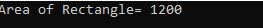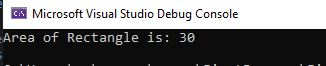﻿ C# Struct - TutorialsTrend
This Angular tutorial helps you get started with Angular quickly and effectively through many practical examples.
`

# C# - Structure

A Struct is also called structure. In C#, a struct is a simple user-defined type that composite a small set of related variables and methods. It is a value type and take place in the stack. C# Struts support access modifiers, constructors, indexers, methods, fields, nested types, operators, and properties.

#### Why use Struct in C#

When you create a small data structures that are often created and destroyed such as coordinates or colors, are defines as structures. Struct can be more effective than classes because they are value types, but they also have some restrictions. Structs, unlike classes, do not have default constructors and cannot inherit from other structs or classes. It is useful if you have data that is not intended to be modified after creation of struct.

#### Structure Declaration

A structure is declared using struct keyword. The default modifier is internal for the struct and its members.

The following example declares a structure Coordinate for the graph.

Example

``````struct Coordinate
{
public int x;
public int y;
}``````

Now access the struct in program.

``````using System;

namespace FirstProgram
{
class Program
{
static void Main(string[] args)
{
Coordinate point;
point.x = 30;
point.y = 40;
Console.WriteLine("Area of Rectangle= "+point.x*point.y);
}
struct Coordinate
{
public int x;
public int y;
}

}
}``````

Output#### Struct Constructor in C#

A struct cannot contain a parameterless constructor. It can only contain parameterized constructors or a static constructor. Let's see another example of struct where we are using constructor to initialize data and method to calculate area of rectangle.

``````using System;
namespace FirstProgram
{
class Program
{
static void Main(string[] args)
{
Point r = new Point(5, 6);
Console.WriteLine("Area of Rectangle is: " + (r.x * r.y));
}
public struct Point
{
public int x;
public int y;

public Point(int x, int y)
{
this.x = x;
this.y = y;
}
}
}
}``````

OutputPrev Next